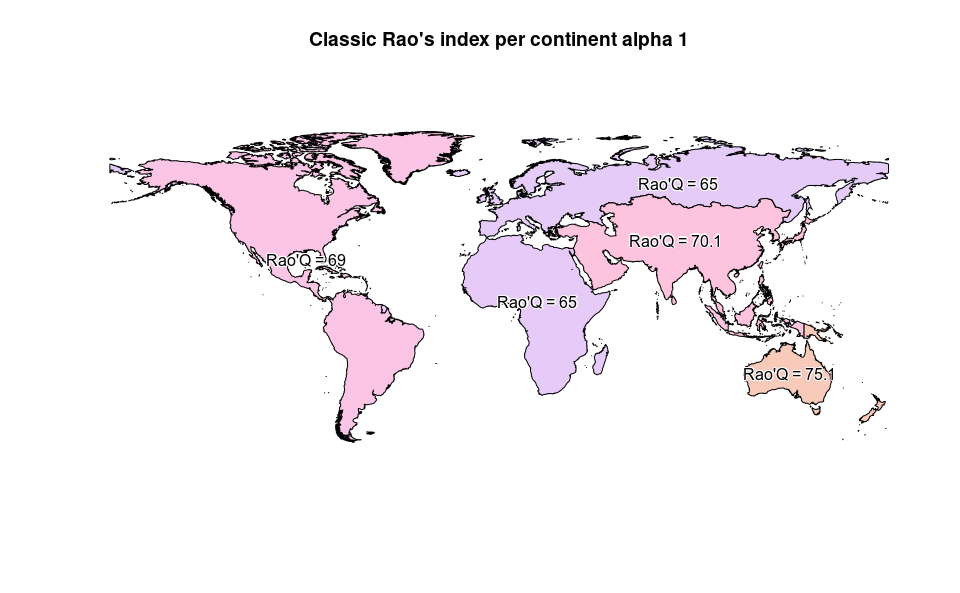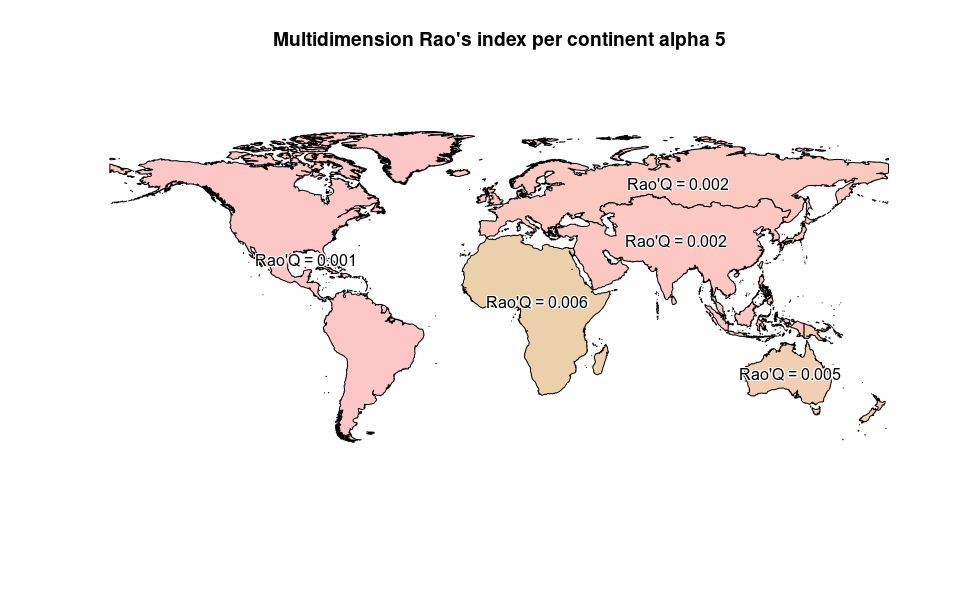# Area-based Rao’s index.

#### 2022-11-22

require(rasterdiv)
require(rasterVis)
require(RColorBrewer)

This vignette uses rasterdiv to derive classic and multidimension area-based Rao’s index from an input vector layer and multiple raster layers.

## Overview

A RasterLayer called copNDVI is loaded together with the package rasterdiv. copNDVI is a 8-bit raster, meaning that pixel values range from 0 to 255. You could stretch it to match a more familiar (-1,1) values range using raster::stretch(copNDVI,minv=-1,maxv=1) .

## Reclassify NDVI

Pixels with values 253, 254 and 255 (water) will be set as NA’s.

copNDVI <- raster::reclassify(copNDVI, cbind(252,255, NA), right=TRUE)

## Resample NDVI to a coarser resolution

To speed up the calculation, the RasterLayer will be “resampled” at a resolution 10 times coarser than original and cut on Africa.

#Resample using raster::aggregate and a linear factor of 10
copNDVIlr <- raster::aggregate(copNDVI, fact=10)
#Set float numbers as integers to further speed up the calculation
storage.mode(copNDVIlr[]) = "integer"

## Compute Area based Rao’s Index

RaoC <- paRao(x=copNDVIlr, area=world, field='CONTINENT', alpha=c(1,2))
#Plot classic area-based Rao's index
plot(RaoC, col=hcl(RaoC$alpha.1*10), main="Classic Rao's index per continent alpha 1") text(RaoC, label=paste("Rao'Q =", round(RaoC$alpha.1,1)), col="black", family="Arial", halo=TRUE)## Compute multidimension area-based Rao’s Index

# The second layers is NDVI/100 (just as an example)
RaoMC <- paRao(x=stack(copNDVIlr,copNDVIlr/2), area=world, field='CONTINENT', alpha=5, method="multidimension", simplify=1)
#Plot area-based RAo's index
plot(RaoMC, col=hcl(RaoMC$alpha.5*10000), main="Multidimension Rao's index per continent alpha 5") text(RaoMC, label=paste("Rao'Q =", round(RaoMC$alpha.5,3)), col="black", family="Arial", halo=TRUE)